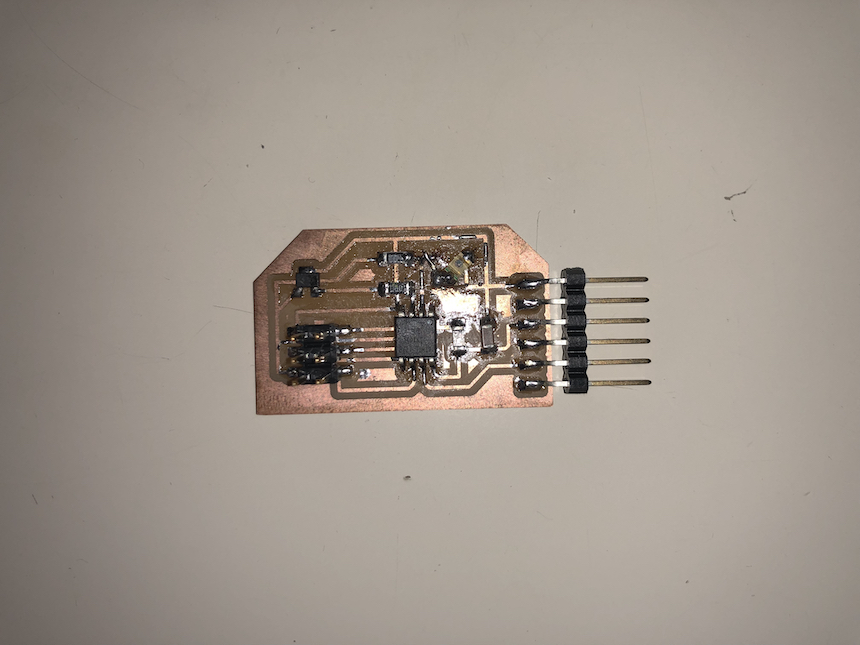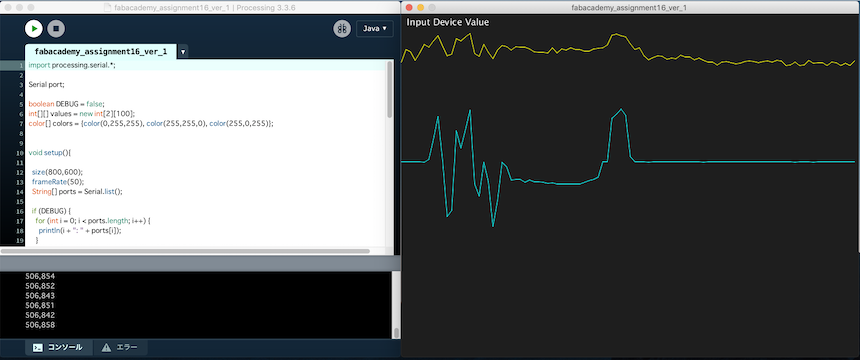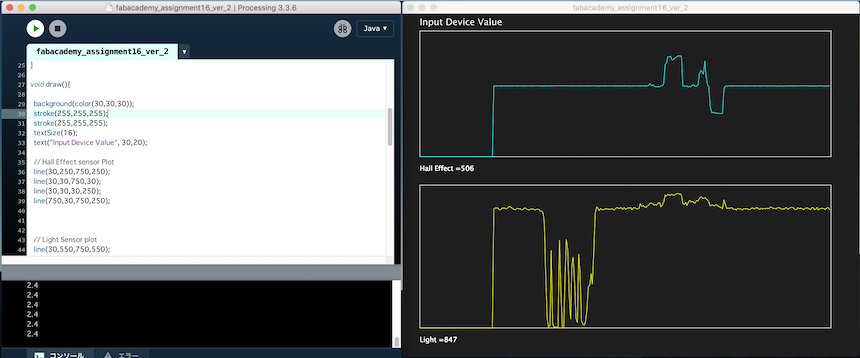# 16. Interface and application programming¶

The topic of this week is about “Interface and Application Programming”. In the class, various kinds of programming languages, frameworks, libraries are instroduced to develop something like software for controlling robots, machines or anything that I would make. The class video is here.

### Assignment¶

• individual assignment:
• write an application that interfaces with an input &/or output device that you made
• group assignment:
• compare as many tool options as possible

In the group assignment, we compare some programming tools. Please also see our group assignment.

### Sensor Data Visualization¶

In the assignment of Input Devices Week, I made an input device that are equipped hall effect and photo transistor.So, in this week assignment, I make an application to visualize the values of two sensor data.I used Processing for developing this visualizing application.

### Simple program for checking serial communication¶

First, I wrote a simple Processing program in order to check the program that receive sensor data via serial communciation. Here is the code of the program.

```import processing.serial.*;

Serial port;

boolean DEBUG = false;
int[][] values = new int;
color[] colors = {color(0,255,255), color(255,255,0), color(255,0,255)};

void setup(){

size(800,600);
frameRate(50);
String[] ports = Serial.list();

if (DEBUG) {
for (int i = 0; i < ports.length; i++) {
println(i + ": " + ports[i]);
}
} else {
port = new Serial(this, ports, 9600);
}

}

void draw(){

background(color(30,30,30));
stroke(255,255,255);
textSize(16);
text("Input Device Value", 10,20);

for (int i=0;i<2;i++){
stroke(colors[i]);
for (int j=0;j<99;j++){
float a = map(values[i][j],0,1023,0,512);
float b = map(values[i][j + 1],0,1023,0,512);

line(8 * j, 512-a, 8* (j + 1), 512-b);
}
}
}

void serialEvent(Serial p){

if (p.available() > 0) {
try {
if (input != null) {
input = trim(input);
String [] value = split(input, ',');
println(value +","+ value );
for (int i = 0; i < 2; i++) {
values[i] = append(subset(values[i], 1), int(value[i]));
}
}
} catch (RuntimeException e) {
}
}

}
```

Here is the result of the program. The program shows the values of two sensor data. It works well.### Line Chart Program.¶

One of the coolest features of Processing is that this framework is very easy to draw graphics. Therefore, it could be very useful for developing something like data visuaklization program. The pixel drawing is very beautiful.

I developed Line Chart program that maps sensor data in realtime with using serial communciation. Here is the code of the program:

```mport processing.serial.*;

Serial port;

boolean DEBUG = false;
int[][] values = new int;
color[] colors = {color(0,255,255),color(255,255,0)};

void setup(){

size(800,600);
frameRate(50);
String[] ports = Serial.list();

if (DEBUG) {
for (int i = 0; i < ports.length; i++) {
println(i + ": " + ports[i]);
}
} else {
port = new Serial(this, ports, 9600);
}

pixelDensity(displayDensity());

}

void draw(){

background(color(30,30,30));
stroke(255,255,255);
stroke(255,255,255);
textSize(16);
text("Input Device Value", 30,20);

// Hall Effect sensor Plot
line(30,250,750,250);
line(30,30,750,30);
line(30,30,30,250);
line(750,30,750,250);

// Light Sensor plot
line(30,550,750,550);
line(30,300,750,300);
line(30,300,30,550);
line(750,300,750,550);

for(int i=0;i<2;i++){
stroke(colors[i]);

for (int j=0;j<299;j++){
float a = 0.0;
float b = 0.0;

float divide = 2.4;
println(divide);

String disp = "";
if(i==0){
a = map(values[i][j],0,1023,250,0);
b = map(values[i][j+1],0,1023,250,0);
line(30+(divide * j),a, 30+(divide * (j+1)),b);
}else{
a = map(values[i][j],0,1023,550,300);
b = map(values[i][j+1],0,1023,550,300);
line(30+(divide * j),a, 30+(divide * (j+1)),b);
}

textSize(12);
disp = "Hall Effect =" + str(values);
text(disp, 30,275);
disp = "Light =" + str(values);
text(disp, 30,575);

}

}

}

void serialEvent(Serial p){

if (p.available() > 0) {
try {
if (input != null) {
input = trim(input);
String [] value = split(input, ',');

for (int i = 0; i < 2; i++) {
values[i] = append(subset(values[i], 1), int(value[i]));
}
}
} catch (RuntimeException e) {
}
}

}
```

The result of the program is here:Here is the Hero shot of the program. It also works well!!!.

### Week 16 Files¶

Processing Programs